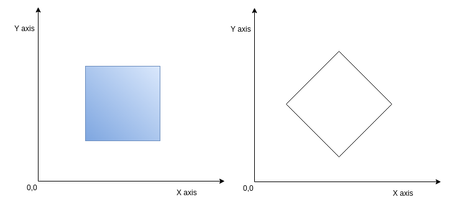# Rotated Squarefaiyaz26Cybernauts'16 National Pr...
Limits 10s, 512 MB

You are given $N$ 2D points, and some query 2D points. For each query point you need to find the area of maximum bounding empty rotated square where the query point is in the center. Rotated square is a normal axis parallel square but rotated by 45 degrees. And empty rotated square means a rotated square which doesn’t contain any point from the given $N$ points. Points can be on the border of the square but not inside. Check the diagram to see what a rotated square looks,If you see the above figure, the left one is normal square, and the right one is rotated square.

## Input

Input starts with an integer $T$ ($T ≤ 5$), denoting the number of test cases. Each case starts with a line containing two integers: $p$, $q$ ($1 ≤ p, q ≤ 100000$), where $p$ denotes the number of points and $q$ denotes the number of query points. Each of the next $p$ lines contains two integers $x$ $y$ denoting the co-ordinate of a point. All the points are distinct. Each of the next $q$ lines contains two integers $x$ $y$ as well. You can assume that the values of the coordinates lie between $0$ and $10^9$ (inclusive).

## Output

For each case, print the case number in a line first. Then for each query point, you have to print the maximum bounding empty rotated square’s diagonal length centered at the query point. It is guaranteed that the answer will always be integer.

## Sample

InputOutput
1
4 2
1 1
100 100
8 9
2 2
0 0
100 100
Case 1:
4
0


For the second query point, you can’t create any rotated square with positive area centered at (100,100) because query point is also one of the given points.

Dataset is huge, use faster I/O.

### Submit